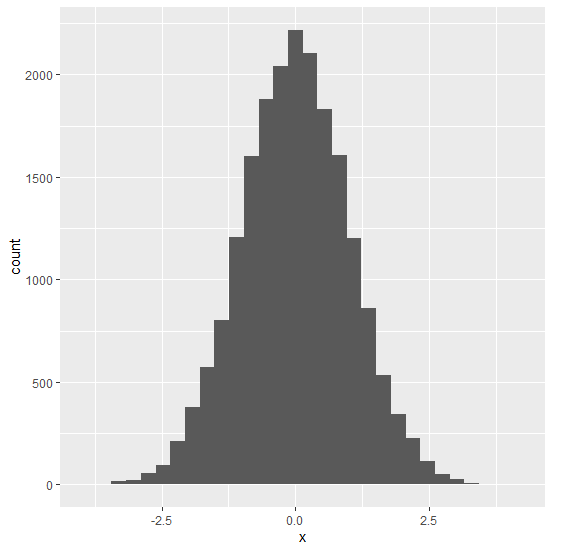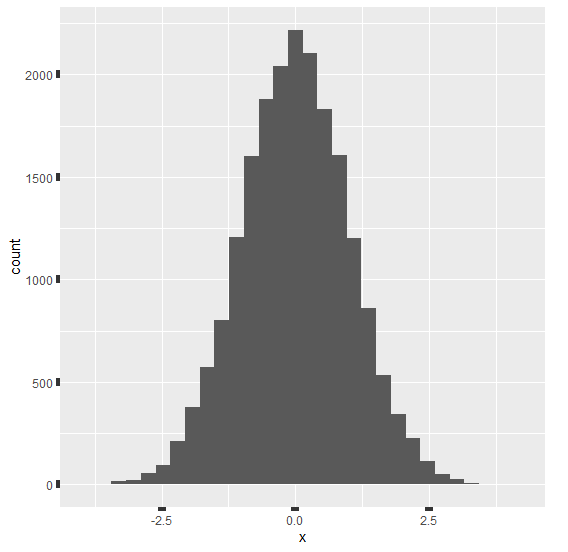# How to increase the axes tick width using ggplot2 in R?

To increase the width of axes tick (both X-axis and Y-axis at the same time) using ggplot2 in R, we can use theme function with axis.ticks argument where we can set element_line argument size to a larger value.

For Example, if we have a data frame called df that contains a single column X and we want to create histogram of X with wider axes ticks then we can use the command given below −

ggplot(df,aes(X))+geom_histogram(bins=30)+theme(axis.ticks=element_line(size=2))

## Example

Following snippet creates a sample data frame −

x<-rnorm(20000)
df<-data.frame(x)
head(df,20)

The following dataframe is created

            x
1  0.92856209
2 -0.46524489
3  0.64398756
4 -1.34904706
5 -1.24865058
6  1.35459661
7 -1.09077357
8 -0.07217955
9 -0.30593246
10  0.04898870
11  0.07062218
12  0.49177492
13 -0.01993569
14 -0.12688569
15 -0.60574285
16 -0.35585400
17  0.77854580
18  0.27782081
19 -0.84785925
20 -0.61172538

To load ggplot2 package and create histogram of x on the above created data frame, add the following code to the above snippet −

x<-rnorm(20000)
df<-data.frame(x)
library(ggplot2)
ggplot(df,aes(x))+geom_histogram(bins=30)


## Output

If you execute all the above given snippets as a single program, it generates the following Output −To create histogram of x with wider axes ticks on the above created data frame, add the following code to the above snippet −

x<-rnorm(20000)
df<-data.frame(x)
library(ggplot2)
ggplot(df,aes(x))+geom_histogram(bins=30)+theme(axis.ticks=element_line(size=3))

## Output

If you execute all the above given snippets as a single program, it generates the following Output −